## Saturday 28 February 2009

### Pancakes on My Mind

There is a nice solution in the comments of my last post by Nate to show that the stack of pancakes never needs more than 2n-3 flips.... but then he adds, "The optimum (or most flippant) arrangement which would require the most flips could be found by working backward from the last flip. " .... hmmmmm, I wasn't sure, so I set about trying his theory will small stacks I probably wouldn't mess up... By Nate's method, there will be a stack of four pancakes with 2(4)-3 = 5 flips necessary... and using his inversion process, 1234, would be the fifth step, so 2134 was the previous, or fourth step, and 3124 the step before that. The second step would be 1324, and the first step would be to 4231, so we must have started at 2431... But it concerned me that I had no proof that the "flip the largest to the top, then flip the whole stack to get it on the bottom" method was ALWAYS the best strategy. For instance, if we start at 2431, we could flip the top three to get 3421. Then if we flip the top two, we would have 4321, and finally flipping the whole stack, we are home to 1234...in only THREE moves, rather than five.

Now the shocker. Not only does the method not always produce the fastest results, the 2n-3 is an upper boundary on the number of flips, but it may not always be the maximum. I think it is for three pancakes, but it seems that for almost any other number of pancakes, you need less than 2n-3 for the most difficult stack.

After investigating furthur, it seems that 2n-3 is almost always more than the number of flips needed. At the Mathworld site, they have a nice table that shows the number of possible ways of stacking the pancakes that require any given number of flips to sort.The table agrees that any stack of four needs no more than four flips, and even a set of five, needs no more than five flips... Which brings me back to Nate's creation. He thought that 31542 would take seven steps to sort, but the Wolfram site suggest it should not need more than five. So today's question, is can you do it in five ??? (or less???).. good luck

## Tuesday 24 February 2009

### A Pancake Day ProblemShrove Tuesday is a term used in several countries for the day preceding the first day of lent. I learned from Wikipedia that " The word shrove is the past tense of the English verb shrive, which means to obtain absolution for one's sins by way of Confession and doing penance. Thus Shrove Tuesday gets its name from the shriving that English Christians were expected to do prior to receiving absolution immediately before Lent begins.

Shrove Tuesday is the last day of "shrovetide", the English equivalent to the Carnival tradition that developed separately in countries of Latin Europe. The term "Shrove Tuesday" is no longer widely known in the United States outside of Liturgical Traditions, such as the Lutheran, Episcopal, and Roman Catholic Churches.  because of the increase in many immigrant populations and traditions since the 19th century. "Mardi Gras" is much more widely-used. The festival is widely associated with the eating of foods such as pancakes, and often known simply as Pancake Day, originally because these used up ingredients such as fat and eggs, the consumption of which was traditionally restricted during Lent."

I found out that some call the previous saturday, "Egg Saturday", and the monday before Shrove Tuesday is known as "Collop Monday" (A collop is chunk of meat or fat), and the cool mathword on the Sunday before is "Quinquagesima Sunday" which means the fiftieth day; Easter then, would be the first day in the string.

So here is the problem... I cooked up a bunch (call it n) of pancakes for pancake Tuesday, but the stack was not as neat as I wanted. I really wanted them to be stacked with the largest diameter on bottom and the smallest on top. I decided I wanted to restack them by inserting the spatula into the stack somewhere under the top x of them, and flip them over on top of the n-x below. Using only this process, what is the maximum number of times I would have to flip cakes to get them in my preferred order, no matter how they had been originally stacked?

Now pass the maple syrup someone, please?

## Sunday 22 February 2009

### Two Nice QuestionsI have talked before about Dave Marain's web site at Math Notations. He has a new contest for grade ten and under that has a wide enough span of problems that it even attracks some middle schoolers. If you teach in the grade eight to eleven range, you might want to drop in, and perhaps participate. He covers some nice stuff.

In particular, he posts a Math Problem of the Day (with a solution) and all the ones I have seen have been really interesting challenges. Good for bright kids who need a challenge that textbooks often don't offer. One of the problems from his last contest illustrates a nice property that too few teacherx and students know about quadratics.
The question was:
" The graphs of y = 2x+3 and y = -x2 + bx + c intersect in 2 distinct points P and Q, where P is on the y-axis. Let V denote the vertex of the graph of the parabola.

(a) Determine all values of b for which the points Q and V coincide.
"

Most students who are at all clever know that the constant term, c, of a quadratic will give the y-intercept, and if you ask them what happens when you change "c", they will tell you it just moves the whole graph up or down without changing the shape. So if the two points intersect on the y-axis, they must have the same constant term.. ie, that the c term of the quadratic must be 3.
When asked what the A term of a quadratic does the usual answer is that it makes the quadratic "narrower." I really don't like this answer for several reasons. I hope students, even those who say the curve is "narrower", realize that it has a domain from negative to positive infinity, and therefore it never gets "narrow". What they mean, I hope, is that it goes up more quickly for larger values of A. and less quickly for A values closer to zero.
When you ask them about B, they are often less certain. They may tell you it moves the vertex (or the graph) left or right, and maybe they can even give a specific distance (someting about h==b/2A) but in fact there is a little more happening than that. In the image above, the red line is the locus being traced by the vertex as the variable b is animated and a is held as -1, while c=3. It looks quite clearly as if it follows a parabolic path with a leading coefficient that is the negative of the original function, and a vertex at the y-intercept. The confirmation of this should not be beyond a good Alg II, pre-calc student.

A couple of days ago Dave also posted a "Problem of the Day" about a regular dodecagon that got me thinking about a problem I haven't worked out yet. A regular Dodecagon for the Greek Impaired student is a twelve sided polygon. Think of a clock face with all the hour marks connected in sequence. What was given in the problem, is that if you draw a chord from any vertex, to the vertex four hours away, the length of this segment squared is the area of the dodecagon. Ok, I didn't know that. The proof was a pretty easy use of pre-calc tools, primarily the law of cosines and areas of isosceles triangles... but ... I wondered, how often does that happen? How often does a chord between two vertices of a regular polygon represent a quadrature of the figure (the square root of its area)... and what if we allowed easy extensions of this...(ie from a vertex to a midpoint of some edge).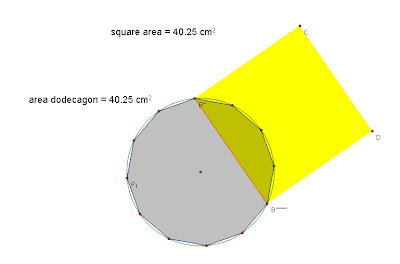Anyway, a big point, Dave's Problems of the Day are pretty good for provoking mathematical thought in students and teachers, so check them out.

## Tuesday 17 February 2009

### Why Bother with Vectors?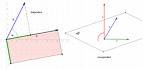The question in the title came from a fellow teacher who had set in on a class in which I was laying the first foundations of what would be several weeks of focus on vectors. Here is part of an answer:

Teacher: So, you took a year of geometry and two years of algebra, right?
student: That's right, Sir (my kids talk nice)
Teacher: So what can you tell me about any two points in space?
Student: Well, they determine a line, I guess..
Teacher: Good, and if I gave you the coordinates of the two points, could you write the equation of the line?

Student: (enthusiastic now, been here, done this)... Sure!

Teacher: Ok, let one be the point at (1, -2, 7)... and the other be at (3, 1, 5)
Student : (totally confused, now).. Huh... what is that.. you have too many numbers...

Ok, that never happened, but if you want it too, just repeat the teacher part with any bright Pre-calc student and I bet they will fill in the details, maybe even with the "Sir" (or Mam as the case may fit)...

I have wondered for a long time at the overemphasis of the slope-intercept form and the almost total exclusion of functions of more than one variable. So for the next few blogs, I'm going to talk about things you can do easily with vectors that seem difficult or impossible without them (or something like them)...

I think, based on my own experience with students, that it takes very little extra time to take a student from the two dimensional slope interecept form to a vector form of equations that will extend to as many dimensions as the student may ever encounter.. (I assume that number will be finite). Some teachers will suggest that there is not any real difference beween my vector form and what is commonly called parametric form of equations, and I agree, as long as all you want to do is write the equation of a line; but I hope to show in the next few days that mixing vectors, matrices, and the traditional equations of planes can quickly expand the level of three dimenisonal tasks that a student can answer.

Today I want to show that writing the equation of a line with vectors is really as easy as using slope intercept, and may actually make more sense in some ways to the students. Before we jump into three space, we might try to win the student over with a two space example..... for example, suppose we want to write the equation of a line though the points (3,1) and (1,-2) in the X-Y plane. First we find the slope, like always... and it turns out to be 3/2 (what do you say to the kid who writes -3/-2)... Then we can use the slope and one point to write the equation as y-1 = (3/2)(x-2) ...(I walked around an Alg I class today as they were being shown this method, and while the rule and an example were on the board, about 1/3 of the student's whose shoulders I peered over had reversed the x and y coordinate values on the subsequent example ...we drill it into them that x comes before y... hmmm)

I think the kid who really knows what is going on, when asked to graph this line will go to the board, mark the point (3,1) (or perhaps (1,-2)) and then count over two to the right, then up three, and make another point. They might repeat this a couple more times, then sketch a line through the points drawn... To most kids, the line goes "over 2, up three". What if we actually let them write it as [2,3] . [after a couple of examples, we might ask the student how long is the line segment between the two points... wait... see how long it takes them to notice that the two legs are right here in the vector) We could define the line as the set of all points (x,y) so that (x,y)=(3,1) + t [2,3] (there is absolutly no reason mathematically to write the point in parentheses and the slope (vector?) in brackets, but I think there is pedagogically). Now imagine that a classroom full of kids had been trained to write the two-dimensional equations as shown, and then we say... hey guys, suppose we want to write the equation of two points in space, with points (x,y,z)... between the two points at (1, -2, 7)... and at (3, 1, 5). I can tell you that in my experience working with Alg II and Pre-calc kids, they automatically extend the method naturally..... almost every one of them... and when I go really crazy, and ask them to write a line between two points in four space, over half the kids in class are wagging hands to get a shot at the board. And when I ask them the distance between the two points, there is almost never a question about whether the Pythagorean method applies... vectors is vectors, baby!

Down the line, I think there are some great modifications. If we develop the practice of writing the slope vector (I call it that becuase they learned "slope" first, but it could easily be called the translation vector) as a unit vector. The variable t suddenly takes on the additional value of indicating the distance away from the original point in the vector direction; substitute in t=3, and you get the point 3 units away..etc. Now we ask them to find the point three-fifths of the way from point A toward point B and they write A + 3/5 [B-A] almost without instruction; and yes, they will know what to do if you ask for it the other way around.

There are some heavy ideas you might want to explore here that you could almost never touch using the traditional equations. Imagine giving your students the coordinates of a triangle in three space and have the students find the point where the medians intersect. Later with dot and vector products, and a little work with matrices to find solutions to systems of equations, we will find the equation of a line along the intersection of two planes, the foot of the perpendicular to a tetrahedron etc.

Someone who is really good at linear algebra could probably point out fifty other little tasks that are easy to do in three-space with vectors and matrices.. (and I would love to hear from you)... But for now, try introducing some vector equations of lines to your kids.. I bet they can pick it up in one class period, and it is a natural companion to parametric equations (which is the area of the curriculum I use to justify the very little time I go off task on three space vectors and matrices)

Stay tuned... Next I'll write use the dot product to find the angle between two lines or segments in space.

## Monday 16 February 2009

### Lincoln and EuclidI wrote once about Linconln's interest in the meaning of "demonstrate" and how it led him to study Euclid. I just came across a rather extended account of the same story posted by John Armstrong at The Unapologetic Mathematician which is worth repeating, so here 'tis:
"I want to point out something about Abraham Lincoln.
It’s said that he carried three books with him, particularly as a young itinerant lawyer. The Bible is a given. A collection of Shakespeare isn’t unexpected, considering his practiced ease with language. But the third? Lincoln studied Euclid, and carried a copy of the Elements. His law partner tells of Lincoln stretched out on the floor, reading geometry in the lamplight.

Lincoln himself explained his interest:
In the course of my law reading I constantly came upon the word “demonstrate”. I thought at first that I understood its meaning, but soon became satisfied that I did not. I said to myself, What do I do when I demonstrate more than when I reason or prove? How does demonstration differ from any other proof?

I consulted Webster’s Dictionary. They told of ‘certain proof,’ ‘proof beyond the possibility of doubt’; but I could form no idea of what sort of proof that was. I thought a great many things were proved beyond the possibility of doubt, without recourse to any such extraordinary process of reasoning as I understood demonstration to be. I consulted all the dictionaries and books of reference I could find, but with no better results. You might as well have defined blue to a blind man.

At last I said,- Lincoln, you never can make a lawyer if you do not understand what demonstrate means; and I left my situation in Springfield, went home to my father’s house, and stayed there till I could give any proposition in the six books of Euclid at sight. I then found out what demonstrate means, and went back to my law studies. "

Thanks Johh.

### The Cheshire Math CurriculumIn a note to the Philosophical Magazine (January, 1846) by Professor J.R.Young of Belfast College, Working with the sum of infinite series he refers to the "evanescence" of xinf when x < 1.. One of the things I love about old Math journals is the beauty of the language used. For those who may not know the word, it means to slowly disappear, to vanish.

I was reminded of this quote recently thinking about the evanescence of many of the ideas once common to the high school curriculum. I recently had a math teacher who teaches Alg I tell me that factoring was not in the curriculum for her students, and she would not be teaching it. I know many others for whom, although it is still in their curriculum, it will not be taught because they don't see a use for it. In a blog a teacher of introductory students in a community college stated, almost smugly, that they had never learned the quadratic formula. The apparent implication, "It is not needed because I don't use it and I'm the teacher."

I recently wrote some blogs about synthetic division, including a few things that some folks had said to their classes "can't be done" and received several notes reminding me that it was a waste of time, because they could just use long division.... BTW, guess what is being de-emphasized in elementary school these days...... evanescence...

Last year I noticed that Descartes Rule of Signs had essentially "evenesced" out of my Pre-calc sequence. The term and the idea are both absent from my new algebra II book, and appears only as the last problem (number 73) in an exercise set in the current Pre-calc/analysis book.

A brief treatment of vectors is still present, but seeming unsteady on legs as wobbly as a punch-drunk fighter. No vector equations (nor parametric if you see them as I do as almost interchangeable) in three space, although there is a unit on two space parametric equations to accomodate a few questions on projectile motion.

For most of the year I squeeze these in as extra items, one this week, one the next, so that by the time we get to this time of the year I can give a full couple of weeks to vectors and planes in three space and my best students will actually be able to write the equation of a line of intersection of two planes; or the foot of the altitude of a tetrahedron from its four coordinates.

It's not in the curriculum, and I have no answer when someone protests that the students will "NEVER have to use that." Maybe they are right..I know that long ago when I was selecting classes in North Side High School in Fort Worth, Texas, I could not imagine any time in my life when I would need to speak French... (guess where I am going back to on Spring Break because Christmas there was so wonderful).. and I can't imagine anyone sitting around doing math, punching buttons on their calculator or ??? and they suddenly think to themselves, "I wish I didn't know how to factor polynomials, and I can't wait to forget how to do synthetic division

## Sunday 15 February 2009

### Weekend in LondonSpent the weekend in London with my beautiful wife. We went down to see a lecture on Pierre Teilhard de Chardin. the French Priest, Paleontologist, and mystic, but the Hilton made us a good deal so we stayed an additional night. We took in the Tate Modern as well, but I admit, the score was Tate, 1, Me, 0... Okay, I just don't get modern art... A huge display that was mostly metal bunk beds all painted blue or yellow with books on the uncovered springs ... sort of "Nightmare at Ikea."...

On the plus side, I like just walking around London. While strolling down Fleet Street I came across St. Bride's church, tucked away down a little narrow lane. Apparently St. Bride's is short for St. Bridget's, and the church is one of the oldest church sites in London, possibly dating back to the sixth or seventh century when the Irish priests came to save the pagen Saxons. The church, like so many others in London, was rebuilt after the "great fire" by Sir Christopher Wren, and is the 2nd tallest spire in the city. The spire (above) was a modification after Wren had finished (the nerve) and is supposedly the inspiration for the modern wedding cake (I know, the sign on the church gate told me so).

While in the neighborhood, I stopped in at the house that Samuel Johnson lived in during much of the time he wrote his English dictionary. While there I was supprised to find a note about a Lord Monboddo who seems to have been onto evolution well before Darwin. 'His lifetime’s work, The Origins and Progress of Language, published in three volumes between 1771 and 1776, was a pioneering work, anticipating, though in some odd ways, later discoveries in anthropology, biology, and zoology. He daringly ignored the current religious-based belief that human language was bestowed fully developed as a divine gift, and instead held that it was something that slowly evolved over a long period of time.

Most remarkable, though, was his observation of the humanoid features of the orangutang, in his day still a little-known primate. Monboddo daringly suggested that the orangutang might be related to humans. Anticipating Charles Darwin by generations, this offhand observation only brought down ridicule. The situation wasn’t helped either by some other strange observations in the book, such as his belief that a race of humans with tails existed somewhere around the Bay of Bengal."

Enough for now, I will return to math, which I know a (very) little more about than modern art, next time.

## Wednesday 11 February 2009

### Don't Write the Law of Sines Upside Down, Please!

Mostly I'm a teach and let teach kind of guy... you do what you think is important, and let me do the same... but sometimes when I see people teach the law of sines... I wonder... DO THEY KNOW?????
The ideas behind the law of sines, like those of the law of cosines, predate the word sine by over a thousand years. Theorems in Euclid on lengths of chords are essentially the same ideas we now call the law of sines. The law of sines for plane triangles was known to Ptolemy and by the tenth century Abu'l Wefa had clearly expounded the spherical law of sines (in 2014 Thony Christie sent a note telling me that "Glen van Brummelen in his "Heavenly Mathematics: The Forgotten Art of Spherical Trigonometry" says the spherical law of sines was discovered either by Abū al-Wafā or Abu Nasr Mansur .  It seems that the term "law of sines" was applied sometime near 1850, but I am unsure of the origin of the phrase (and if you have a reference, please advise).A simple proof of the law of sines begins with a triangle, ABC, inscribed in a circle with radius R. A diameter is drawn with one endpoint at A terminating at D and the right triangle ADC is created. Using the right triangle definitions of Sine, we see that sin (ADC)=AC/AD.

Because Angles ABC and ADC are both inscribed angles cutting the same arc, they have equal measures, and therefore equal sines. By substitution then we get sin(B)=AC/AD and since AD is a diameter equal to 2R , we may also write sin(B) =AC/2R . Now if we adopt the modern convention of calling the side AC opposite angle B, side b, we can rewrite this as sin(B)= b/2R. With one last algebraic manipulation we exchange the positions of sin(B) and 2R to get 2R= b/sin(B) [Thanks to Joshua Zucker who reminded me that the radius of the circumcircle is usually capitalized, with r used for the radius of the incircle]. Since the choice of angle B was arbitrary, we could show that the same holds for each side and opposite angle pair, producing the typical high school textbook theorem below.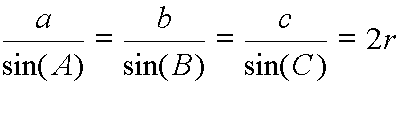(as per Joshua Zucker's note below, that r should probably be R since that is more commonly used for the radius of the Circumcircle, with r used for the incircle. I leave it rather than having to scrabble together a new graphic)
Addendum: [ I still get emails and comments that seem not to realize that by writing the sides on top you can reduce the entire thing to a single geometric relationship.  For any triangle, the ratio of a side to the sine of the opposite angle is always equal to the diameter of the circle which circumscribes the triangle.  Perhaps I should have added this earlier.]

I am frequently amazed to see this theorem presented in math texts without the "=2R" which seems to give it visual or geometric life. It is especially curious since the property dates back to Ptolemy. I get even more frustrated when it is presented with the Angles on top, thus destroying the geometric meaning. I can't think of a good reason for doing that, but if you consciously do it the other way for some reason, I would love to hear it.

As a footnote, in spherical triangles it is customary to work with a sphere of unit radius, thus allowing the sides to be expressed in radian or angle measure as well as the angles. Since all great circles have length 360 degrees, we may express the length of a side by the fraction of a complete great circle it occupies. With this convention, the spherical law of sines states that in a spherical triangle with sides a, b, and c and angles A, B, and C, it is true that

$\frac{sin a}{sin A} = \frac{sin b}{sin B} = \frac{sin c}{sin C} = \frac{sin a sin b sin c}{6 Vol(OABC)}$

That is the ratio of  the sin of any side to the sin of its opposite angle  the product of the sines of the sides over six times the volume of the tetrahedron formed by the center of the sphere and the points A, B, and C.

According to Ubiratàn D'Ambrosio and Helaine Selin, the spherical law of sines was discovered in the 10th century. It is variously attributed to al-Khujandi, Abul Wafa Bozjani, Nasir al-Din al-Tusi and Abu Nasr Mansur.
Ptolemy knew the formula  for the planer law of sines and something like the angle addition formula but he expressed them in terms of chords of arcs, not sines of angle.  The half chords, or sines, were introduced by the Hindu mathematician Aryabhata around 500.
The spherical law of sines was first presented in the west by Johann Muller, also known as Regiomontus,in his De Triangulis Omnimodis in 1464. This was the first book devoted wholly to trigonometry (a word not then invented). David E. Smith suggests that the theorem was Muller's invention. The word trigonometry, by the way, seems to have been the creation of Bartholomaus Pitiscus, who used it in the title of a book, Trigonometriae sive de dimensions triangulorum libri cinque in 1595. Among other things the book includes a demonstration of the law of sines and the law of cosines. I find it highly unusual that the first use of a word would be in the title of a book.

As a second footnote, it may be of interest to teachers and students that the use of the unit circle was "unknown much before 1800". I found that out in an article on "Benjamin Banneker's Trigonometry Puzzle" by Florence Fasanelli, Graham Jagger, and Bea Lumpkin that appeared in the MAA online magazine Convergence. Unfortunatly the magazine is no longer free on-line. Older trig tables gave the measurements for the sine, tangent and secant on a circle of very large radius (van Schooten used 10,000,000) rather than on a circle of radius 1, as we do today. Thus, the sin 90°, also called the “total sine” was given as 10,000,000, and the sine of 45° was 707,107 and not 0.707107, as we would use today. Anyone using these tables would use rules of proportion to make any necessary conversions.

Next I hope to talk about Descartes Rule of Signs.. (which has almost completely disappeared from my current textbooks).

## Tuesday 10 February 2009

### The Ultimate Math Geek Clock, and a Joke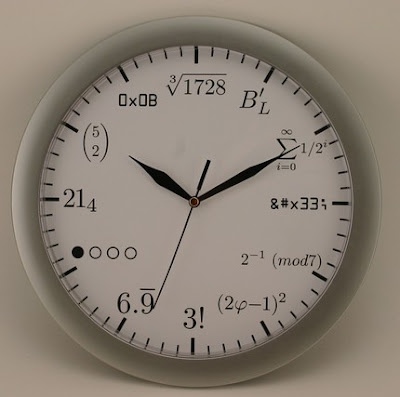I had several blogs awhile back about problems involving clock hands, and today I came across the utimate math clock, above.

As long as we are celebrating math geekdom, here is a recent math geek joke at Foxtrot. Enjoy!

### Solution to Al's Right Triangle Problem.

A right triangle has a perimeter of 60 and the altitude to the right angle is 12. Find the area

When I first heard this problem I recognized the 60 and 12 as simply five times the values of the same measures for a 3-4-5 triangle and so I knew the answer, but also knew I could not answer the question in general. After spending several days seeming to go around in circles, I asked for a suggestion on a math problem solving site I belong to. With the help of the clue I managed to come up with the general solution. So Al, here it is:

If we let the legs of the right triangle be a and b, and let c represent the hypotenuse, then we can start with P=a+b+c... and squaring both sides gives P2 = a2+b2+c2 + 2ab + 2ac + 2bc

By the Pythagorean theorem we know that a2+b2=c2 so we can rewrite that as P2 = 2c2 + 2ab + 2ac + 2bc

Since a and b are the legs of a right triangle, the area, A = ab/2 or 2A= ab and so we replace that in the equation as well... P2 = 2c2 + 4 A + 2ac + 2bc

It was at this point that I seemed unable to make any furthur progress, but finally realized that by factoring I could rewrite 2ac + 2bc as 2c(a+b) which is also 2c(P-c) = 2PC - 2c2, so the equation could be written as P2 = 2c2 + 4 A + 2Pc-2c2 which simplifies to just P2 = 4 A + 2Pc.... So close, and yet it still avoided me... then I realized that since c was the hypotenuse, 2 A= cH (where H is the altitude to the right angle) and so c = 2A/H...

With this substitution I had P2 = 4 A + 4AP/H. The original question could be solved by plugging in values at this point and solving for area, but I wanted to write it out as a general solution, so factoring out the A...P2 = (4 + 4P/H ) A and then dividing by the 4+4P/H producedNext I want to talk about the Law of Sines and why it should NOT be written upside down. See ya' soon.

## Monday 9 February 2009

### A Blog of Two Problems (with apoligies to C. Dickens)

One of my students sent me a nice mathematical puzzle from The Number Warrior by Jason Dyer from the US Puzzle Championship in 2001. The origninal problem was stated this way, "
You have a set of five numbers. When adding together each of the ten possible pairs, you get the following sums:

0, 6, 11, 12, 17, 20, 23, 26, 32, 37

What are the five original numbers?"

I am going to give the way I solved this below so don't read on too much more if you want to work this out by yourself. After the solution I have a couple of variations that occurred to me that I don't have worked out yet, but first I wanted to post a problem Al Harmon sent me from Mr. Milanovich, a teacher at Edgren HS where I used to teach in Misawa, Japan

A right triangle has a perimeter of 60 and the altitude to the right angle is 12. Find the area. ...

I actually was stumped by this problem and had to buy a clue, so if you want the clue, I will put it in the comments, and I will give a specific and general answer to this in my next blog.

Now for the five number problem above.. It is obvious from the fortuitous zero as one of the sums that one of the numbers is negative, and that it is the opposite of the smallest of the four positive values that remain, otherwise there would be one of the sums that was negative.

The next big jump was a statistics idea. I keep trying to remind my students that the mean of the sampling distribution is the same as the mean of the population. A sampling distribution is the set of all the possible measures you could get by taking every possible set of n values from the population. The numbers above represent the sampling distribution of sums from samples of size two. If we add up all ten numbers we get a total of 184, so the average of these "samples of size two" is 18.4; from which we can conclude that the average of the five original numbers is 9.2, and so they must total to 46. But the smallest two add up to zero. From this we can extract that the three largest (call them a, b, and c) add up to 46 also. We know that the two largest add up to 37, so 46-37=9 must be the middle number of the five. At this point I assumed that the 32 represented a+c, the sum of the first and third largest numbers, so if I subtracted 9, I would get the largest number, 23. If that were correct, I would be left with 23+b=37, so the second largest number must be 14. Now looking at the second smallest total, 6, I figured that it had to be the sum of the middle value, 9, and the smallest number. Subtracting 9 from 6 gave -3 for the smallest number, and 3 for its additive opposite.

I then had {-3, 3, 9, 14, 23} for the solution set, and needed only to check that they produced ten distinct sums as given above.

Variations and extenstions:

It came to me as I worked with this problem that it was not necessary for a collection of five distinct numbers to produce ten distinct sums when paired. for example if the set included 1, 3, 4, 6 we would have two sums of 7. It occurred to me to wonder first, what is the probablilty that a set of five distinct integers had ten distinct sums when paired in every possible way. To be answerable, the problem must have some domain for the original values, so I would assume 1-30 would be good for a start. It would seem as if the probability grew as the domain interval grew wider, but I have not actually calculated that out yet. I have not worked an answer for this out yet, and in truth, do not yet have a good idea for an attack other than writing a program to count them.. Anyone who has suggestions (or an answer)... please respond.

I also wondered aobut the probability that any increasing sequence of ten numbers being the sums of five distinct numbers. I am also sort of lost and where to attack this problem, but think it must have something to do with differences in the sums. Again, if you have an idea about a way to attack this, please advise.

Ignore this, it is just a test to see if the equation editor on google will copy to format## Friday 6 February 2009

### Synthetic Division When the Linear Coefficent is NOT One

I was hoping this would be the last one on synthetic division, I have a problem I promised Al Harmon from Misawa, Japan I would answer, and I wanted to talk about the Law of Sines (and how much I hate when people write it upside down)... So I will defer the responses to those of you who asked about the history of synthetic divison for a later day. Today I wanted to answer the challenge to post the way to divide by ax+b without factoring anything out first.

Apparently my ex-students never throw away their notes hoping to catch me if i ever do something a different way, as I did a couple of days ago with dividing both divisor and dividend before I did the division so as to eliminate the leading coefficient of the divisor..... so in case there are other teachers who teach this out there...here is a modified synthetic division approach that will work for 3x+5 and such and still give the right remainder...

I will use the same example 6x2 + 16x + 16 by 3x+5 . To accomodate the 3 in the leading coefficent, a new line is added below the usual bottom line. After each column is summed, (except for the remainder) the result is divided by the linear coefficient, in this case 3.Notice that after we add the first two numbers in a column, we divide that sum by three to get the actual value of the quotient for that term.... but we do NOT divide the remainder.

I also wondered (I had never tried it) if you could do the same, or something similar with the higher degree divisors. I had previously divide x5+ 6x4+4x3-39x2-122x-120 by the cubic x3+3x2-10x-24, so I thought I would try 6x5+ 6x4+4x3-39x2-122x-120 by the cubic 2x3+3x2-10x-24, which Should give a quotient of 3x2 - 3x/2 +77/4 with a remainder of 159/4 x2 + 69x/2 + 342 and see what happens.....Once more we create an extra line to divide by the coefficient of the term with the highest degree (in this case 2) and proceed as we do in the non-linear cases by shifting over one column as we go down the list of coefficients (or the negatives of them, actually).And once more, the result emerges rather effortlessly... Ok, we have some way ugly fractions in there... but you would get them even with all the mess you create with long division...
Call that a wrap...

## Thursday 5 February 2009

### Synthetic Division for Derivatives of Polynomials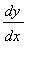Questions, questions, questions... It seems like synthetic division has struck a cord..and I get responses asking or telling me that
a) No one should teach synthetic division;
b) I didn't show you the way to divide by linear terms like 3x-5 directly, and I had shown someone in my class a few years ago (and they remembered,... "thank you, math Spirits)...
c) I should have shown you how to find the derivative like I showed their calculus class (not sure how far back that was..I've done it a couple of times)

My answer to a) is, "If you don't want to teach synthetic division, don't; but please give me the freedom to make that choice for myself."

I will try to answer b in my next blog (soon...)

That leaves c) so today I will illustrate that it is almost as easy to find the value of the derivative at a given value using synthetic division as it is to find the value of a function.

I will use the simple f(x) = x3 - 3x2 + 6x - 7 and we wish to evaluate f(2) and f '(2) ...
From the factor-remainder theorem we know that if a polynomial f(x) is divided by x - b, the remainder is f(b)... so we can simply find the value by synthetic division as below.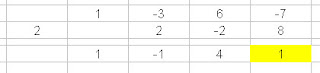From this we see that f(2) = 1... but how does that help us find the derivative... Calculus teachers know that f '(x) = 3x 2 - 6x + 6 , and nothing like that seems to pop out of what we see above... and if we evaluate that for x=2 we get 6... but where is it in the synthetic division?

Patience,... one more run... now evaluate the quotient of the above problem at 2 again....andBehold, as Brahmagupta supposedly wrote.. the remainder of this second division is the first derivative...... and I know your heart is thumping in your chest, wondering, wishing.... what if we did it again, would it be,........ could it be?

Oh, Yeah... and now the descent through the derivatives in similar form is probably clear...

SO WHY does that work?

Let's walk through the second part using some pronumeral value x=a instead of a numberNotice that in the place where we expect the remainder, we see that we get the evaluation f(a)... and when we continue down, you can see the derivative terms accumulate as the division works across to finally reveal, that f '(a) does indeed become 3a2 -6a + 6...

I love math.... You just don't have clever things like that pop out in any other discipline...
For those who would like a slightly more technical explanation, her is one from William Rose:

Write P(x) = (x-a)Q(x) + r
By Remainder Theorem, this is:
P(x) = (x-a)Q(x) + P(a)

P'(a) = lim as x--> a of [ P(x) - P(a) ]/(x-a)
= lim as x--> a of [(x-a)Q(x) + P(a) - P(a)]/(x-a)
=lim as x--> a of Q(x)
=Q(a)

So the theorem says that the quotient after dividing P by a, evaluated at a, is the derivative of P at a. This seems totally transparent when you look at P(x) = (x-a)Q(x) + P(a) in that form. Q(a) is the rate at which P is changing near x =a.﻿

## Tuesday 3 February 2009

### More on Synthetic Division

I wanted to follow up a few things I didn't make as clear as I might have yesterday; first the question about the leading coefficient, then the extension to dividing by a polynomial of higher than the second degree, and the remark about dividing by "breaking up a cubic"

First the question about the leading coefficient.... It doesn't matter what the leading coefficient of the dividend is, but the leading term of the divisor must have a coefficient of one...That is the same as with dividing by a linear factor...... but you can reduce the problem to an equivalent division by dividing out that coefficient...(wow, that was a mouthful.. so here is an example, using the easier linear factor )
Supppose we wanted to divide 6x2 + 16x + 10 by 3x+5.. We need to turn the 3 in 3x+5 into a one, so we divide to get x+5/3.. but this is like simplifying fractions(think of 6x2 + 16x + 10 as the numerator and 3x+5) as the denomiantor) we have to divide both the top and bottom by three to keep the equality, so we also divide 6x2 + 16x + 10 by three, and our new problem is to divide 2x2 + 16/3 x + 10/3 by x + 5/3Keep in mind that if there is a remainder, it will not be the same in the simplified problem as in the original.. If we add 6 to the constant term of the previous problem we get 6x2 + 16x + 16 by 3x+5. Eliminating the leading term of the divisor we get 2x2 + 16/3 x + 16/3 by x + 5/3 and now when we do the synthetic division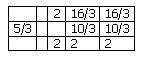we get a remainder of 2, instead of six.... ahhh, but we divided both terms by three, so our true remainder is 3x2=6...Keep in mind that one of the things synthetic division does is give us f(2) for a function (the dividend) of x.. If we divide f(x) by three, the value at any point (and hence the remainder on division) is going to be 1/3 of what it would have been otherwise.

And now... the higher degree issue, which seems to work out quite nicely... If we wanted to divide by a cubic we just add three lines, and continue to move the products over one column.. Here is an example dividing x5+ 6x4+4x3-39x2-122x-120 by the cubic x3+3x2-10x-24... note that we still take the negative of each coefficient of the divisor.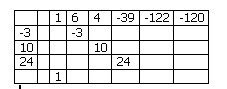As we continue we see that the quotient is x2+3x+5 with no remainder...Finally for the cryptic remark about "breaking up a cubic"... In the last problem, if we had wanted to, and if we recognized that x3+3x2-10x-24 could be factored into (x-3)(x2+6x+8), we could have done the last problem in steps. If we divide by one factor and then by the other, we will stil get the same result (although there is still need to pay special attention to remainders). In arithmetic we can divide 24 by 6, or we can divide it by 3, then divide the answer by 2... In the same way we can do the synthetic division in two steps.. I decided (no special reason.. ) to divide by the linear term first.. then the quadratic, as shown here.## Monday 2 February 2009

### Synthetic Divison by a Quadratic

I was sitting in a school improvement meeting paying less attention than I ought to, and started trying to figure out how to figure out the topic above. What is below is my way.. I tried to find something about this on the internet, but only found sites that deal with linear factors, and some of them said that what I have done was not possible (I hope they are wrong)... Then when I came home I found a post on the AP Calculus Discussion site that asked how to do this very thing, with a citation for an article in the Mathematics Teacher, (March, 1980 journal (hopefully the archives go back that far), there is an article called "Synthetic Division for Nonlinear Factors" ...thanks to Lisa Lewis) that describes the process. Unfortunatly I can't get that on-line.

So here is how I did it.. I made up a problem involving a fourth power polynomial, and a quadratic divisor(A clever student could break this divsor into two linear terms and do the same in two divisions... IF he is careful about remainders, but pretend I had been clever enough to use a irreducible quadratic for the divisor)

Divide x4 + 8x3+ 15x2 + 4x + 1 by x2 + 3x + 2, using synthetic division:

The dividend is written out in the usual order with the coefficients (including any missing zeros)... and to the left two columns with the negative of the B term (3 and the C term (2) as shown below... notice that I use the -B term above the -C term.. and as usual, the bottom row will be the quotient.The first step is still to bring down the leading coefficient.. from there there are two multiplications: the product of the -B term and the number in the bottom row go in the row with -B and the column with the last bottom number... The product of the -C term and the last bottom number will go in the column one to the right of the last bottom number. Note the locations of the -3 under the 8, and the -2 under the 15...Then we add up all the values in the second row to get a new bottom value.We continue this process, always keeping the product of the -C term entered one to the right of the last bottom number.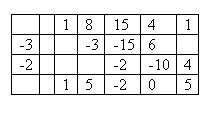Since we are dividing a fourth degree polynomial by a second degree polynomial, the answer will be of the second degree, and the last two cells on the bottom represent a linear remainder. In this case the quotient is x2 + 5x -2 and the remainder is 0x + 5... You can check the solution by multiplication.

Big Idea..... Gauss proved that all polynomials with real valued coefficients can be factored into a product of linear factors (like x-a) and irreducible quadratic factors (qudratics that don't have real roots, and hence can't be factored over the reals). So we can factor any real valued polynomial with nothing bigger than quadratic division....

But what if we have a seventh power polynomial and want to divide it by a cubic and we don't want to do it without breaking the cubic down... can it be done...?

OK, I don't know, but if I get a free moment in the next 24 hours, I think I will try extending this method to a third term and see if I get lucky...

Ok, so the remark about "breaking the cubic down" was not clear, so I will try to cover that in a day or two..... HEY, I have five classes to prep..give a guy a break...

### Archimedes and the Square Root of ThreeSnow here in my part of UK, so I stayed warm indoors reading other math blogs and some old journal articles...
Came across a couple of nice ones, but wanted to talk about one in particular.

Mark Dominus, atThe Universe of Discourse wrote a nice article (actually two of them) about Archimedes and how he might have obtained his fractional approximations to square roots, such as his use of 265/153 for the squre root of three that seem to confuse math historians.

He points out that Historians seem to treat it as if it must be some obscure and difficult approach that Archimedes had never revealed. W.W. Rouse Ball writes, "It would seem...that [Archimedes] had some (at present unknown) method of extracting the square root of numbers approximately." and Sir Thomas Heath writes, "the calculation [of π] starts from a greater and lesser limit to the value of √3, which Archimedes assumes without remark as known, namely 265/153 < √3 < 1351/780. How did Archimedes arrive at this particular approximation? No puzzle has exercised more fascination upon writers interested in the history of mathematics... "

So why wouldn't any of these great mathematicians think that probably someone in ancient Greece thought to make two lists and compare when they were close. One list of the squares (we know there are Babylonian tablets with columns of squares of numbers well back before Archimedes)..and one a list of them mutliplied by three (not a difficult task... In fact they could be prepared (as almost every Egyptian scribe must have known) by adding the sequential odd digits to get the squares, and three times these same odds to get the table of 3n2. Then you could just scroll down the list to find one in each list that was approximatly equal... Here is a list created quickly using Excel with two easy candidates for rough approximations marked in colored cells.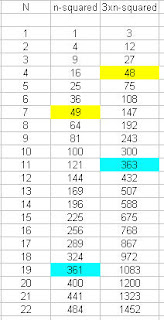If 48, which is 3 (42) is about the same as 72. then by division we know that:Mark also offers a second alternative. Perhaps a master of patterns like Archimedes noticed that as he looked down the list he saw that n2 was approximatly equal to 3p2 when for the following values of n and p;
n = 2....5.....7....19....26....71....97
p = 1....3....4....11....15....41....56

Do you see the pattern? Mark points out that 2+5=7 and 2x7 + 5 = 19... and then 19+7=26 and 2x26+19=71...
for the other string the same thing 1+3=4, and then 2x4+3=11. Each row continues by alternatly adding the last two numbers and then double the last plus the one before... A pattern that would be easily extended as far as he wished to take it; and the next fraction from the pattern are 265/153 which, when squared, gives 2.99991 (pretty close) but the next number is 362/209, which squares to approximatly 3.00002.... Now my question.. why didn't Archimedes use this for the upper bound instead of 1351/780... which follows the lower bound of 989/571 in this sequence

Perhaps the clever thing Archmedes did that baffled modern math historians is that he still had an eye for arithmetic.

## Sunday 1 February 2009

### Superbowl StatisticsThe picture above is the graph (in a sense) of a statistical distribution...actually of at least two.. I came across this on the Statpics blogsite, which is quickly becoming a favorite.
Some really clever uses of illustrations for statistical reasoning.. check it out.

And as long as I'm pimping other peoples blogs, and talking about the dreaded (I really hate the) super bowl, Check out Dave Marain's Math Notations blog where he is asking, "Was the First super bowl more or less than a billion seconds ago?" and making some other quick comparisons about a billion (American, not British)